### Home > CC4 > Chapter 10 > Lesson 10.1.4 > Problem10-69

10-69.
1. For each diagram below, solve for x. Explain what angle relationship(s) you used. Homework Help ✎

1.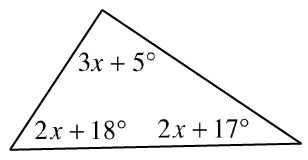2.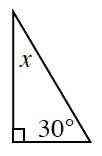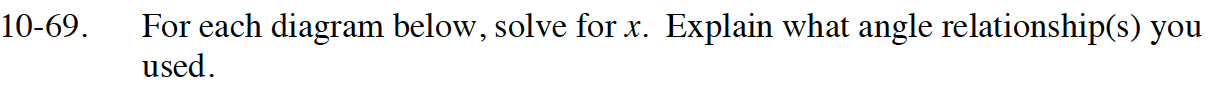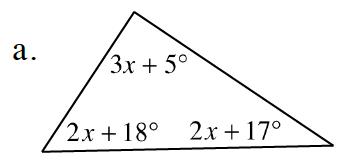Review the Math Notes box in section 7.1.2

Since the angles of a triangle add up to 180°, set up an equation using all three angles.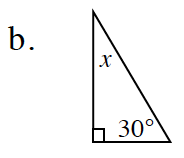x = 60°